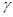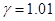Chapter 28, Problem 7PE

Chapter
Section
Textbook Problem

If relativistic effects are to be less than 1%, thenmust be less than 1.01. At what relative velocity isTo determine

The relative velocity whenγ=1.01 If relativistic effects are to be less than 1%, thenγ must be less than 1.01.

Explanation

Given info:

c=3×108 ms1

γ1.01

Formula used:

For time duration for view in the laboratory calculation, the relation between time and

Relativistic factor is

γ11v2c2

Velocity can

vc11(γ)

Still sussing out bartleby?

Check out a sample textbook solution.

See a sample solution

The Solution to Your Study Problems

Bartleby provides explanations to thousands of textbook problems written by our experts, many with advanced degrees!

Get Started